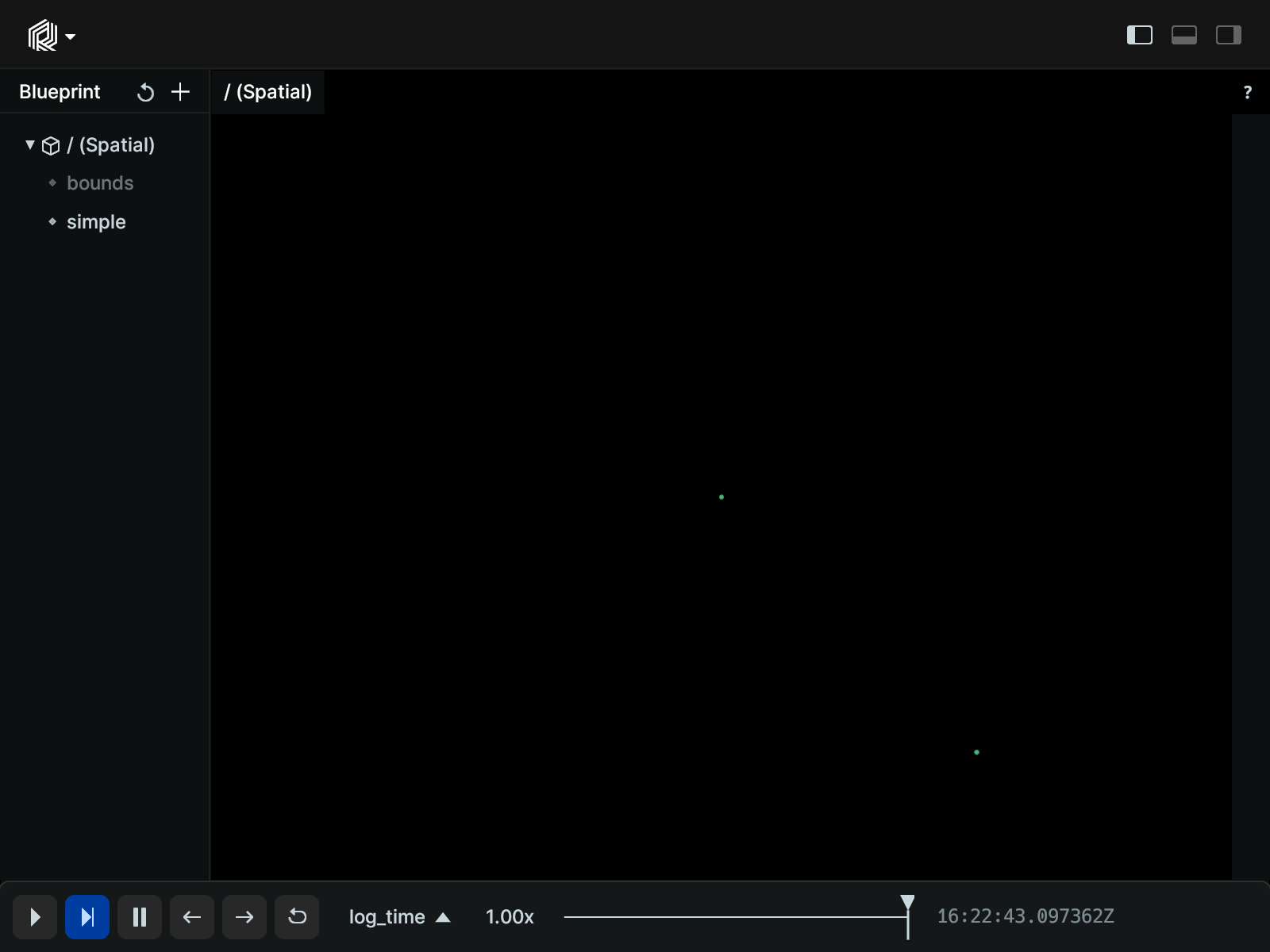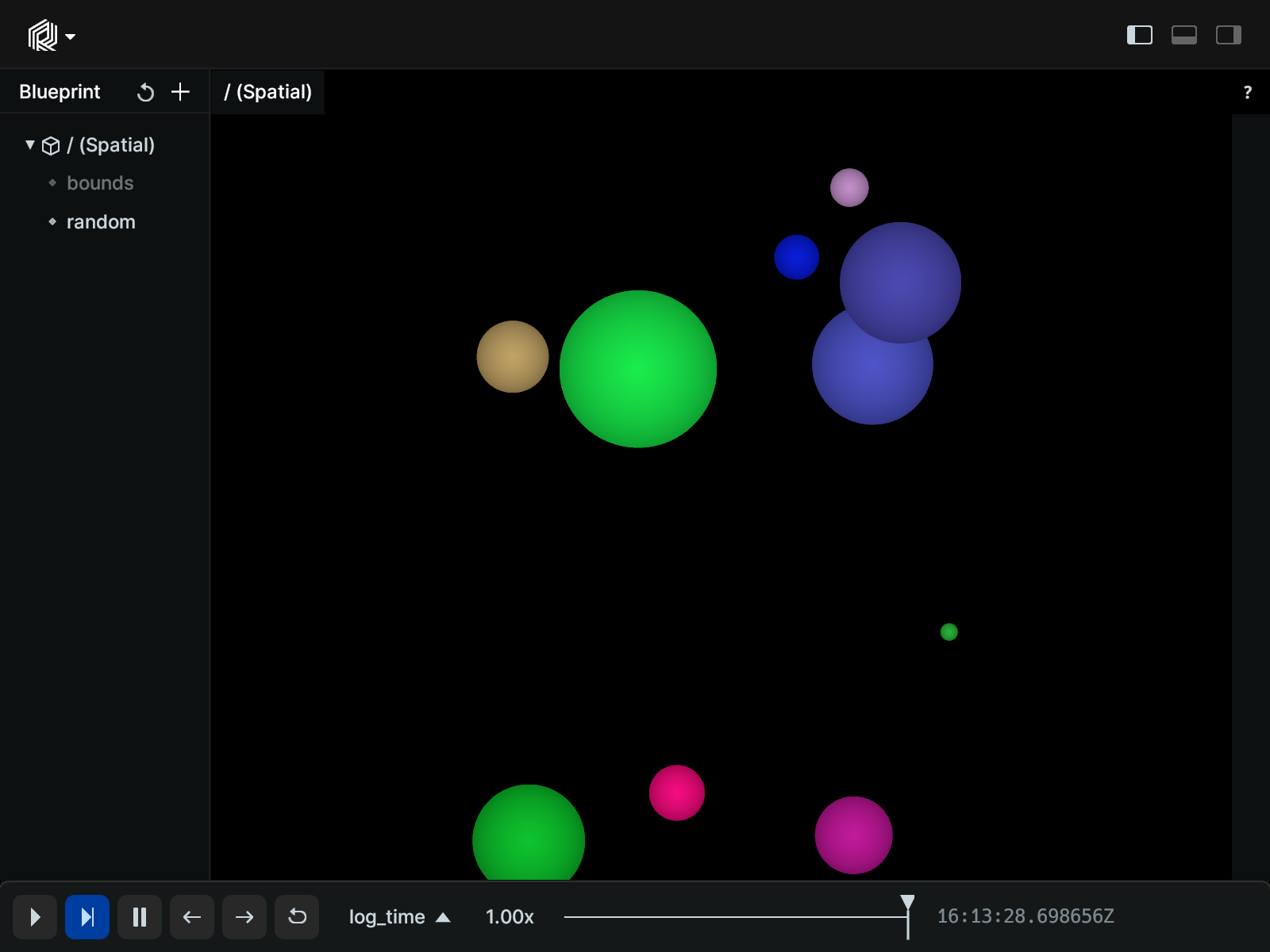# Points2D

A 2D point cloud with positions and optional colors, radii, labels, etc.

## Components

Required: `Position2D`

Recommended: `Radius`, `Color`

## Examples

### Simple 2D points

``````"""Log some very simple points."""
import rerun as rr

rr.init("rerun_example_points2d", spawn=True)

rr.log("points", rr.Points2D([[0, 0], [1, 1]]))

# Log an extra rect to set the view bounds
rr.log("bounds", rr.Boxes2D(half_sizes=[2, 1.5]))
``````### Randomly distributed 2D points with varying color and radius

``````"""Log some random points with color and radii."""
import rerun as rr
from numpy.random import default_rng

rr.init("rerun_example_points2d", spawn=True)
rng = default_rng(12345)

positions = rng.uniform(-3, 3, size=[10, 2])
colors = rng.uniform(0, 255, size=[10, 4])